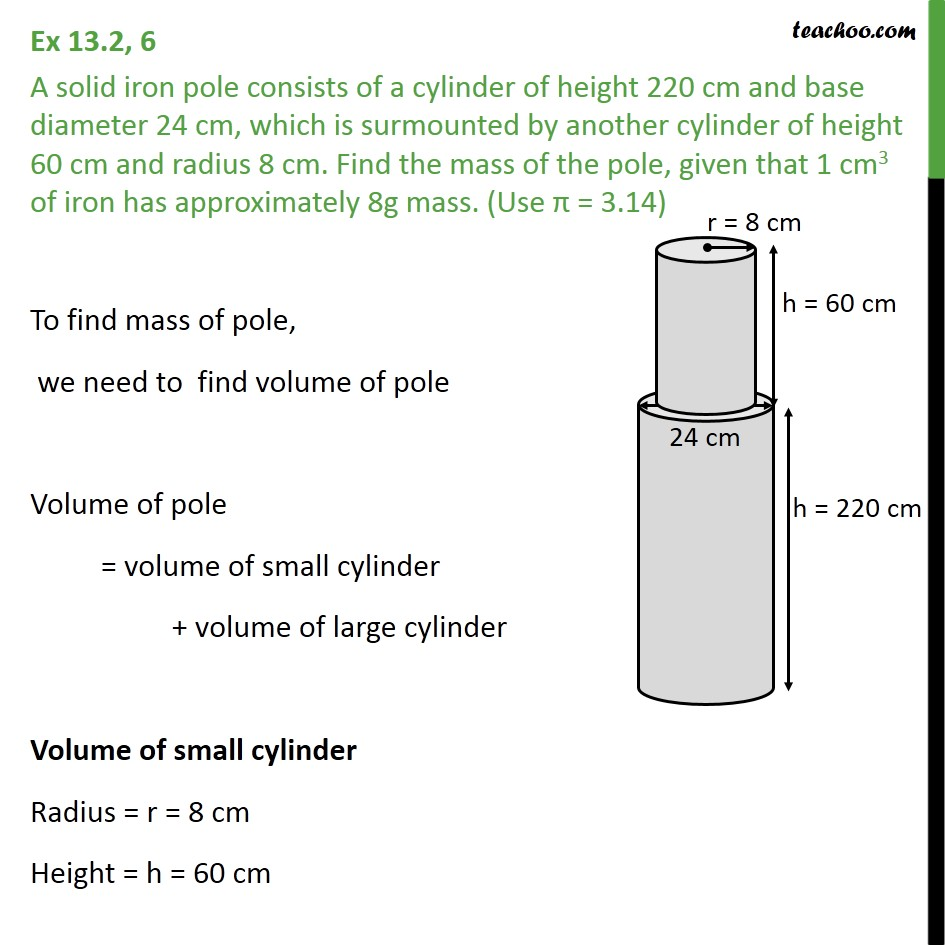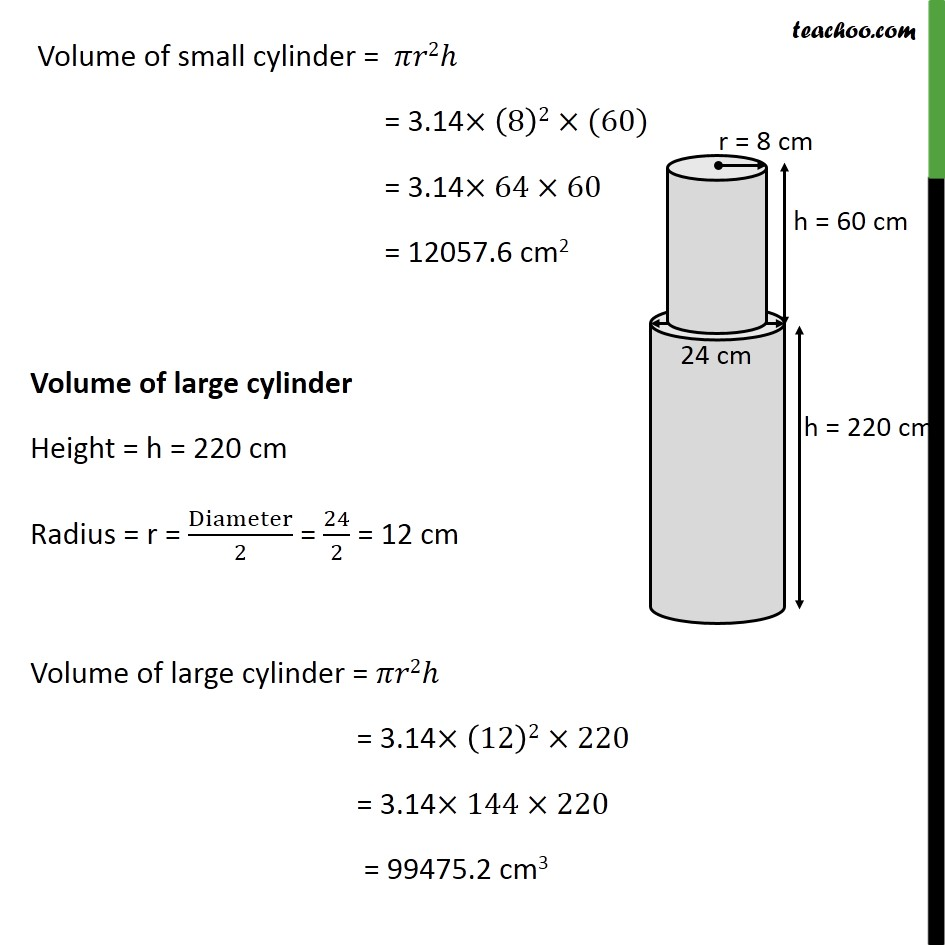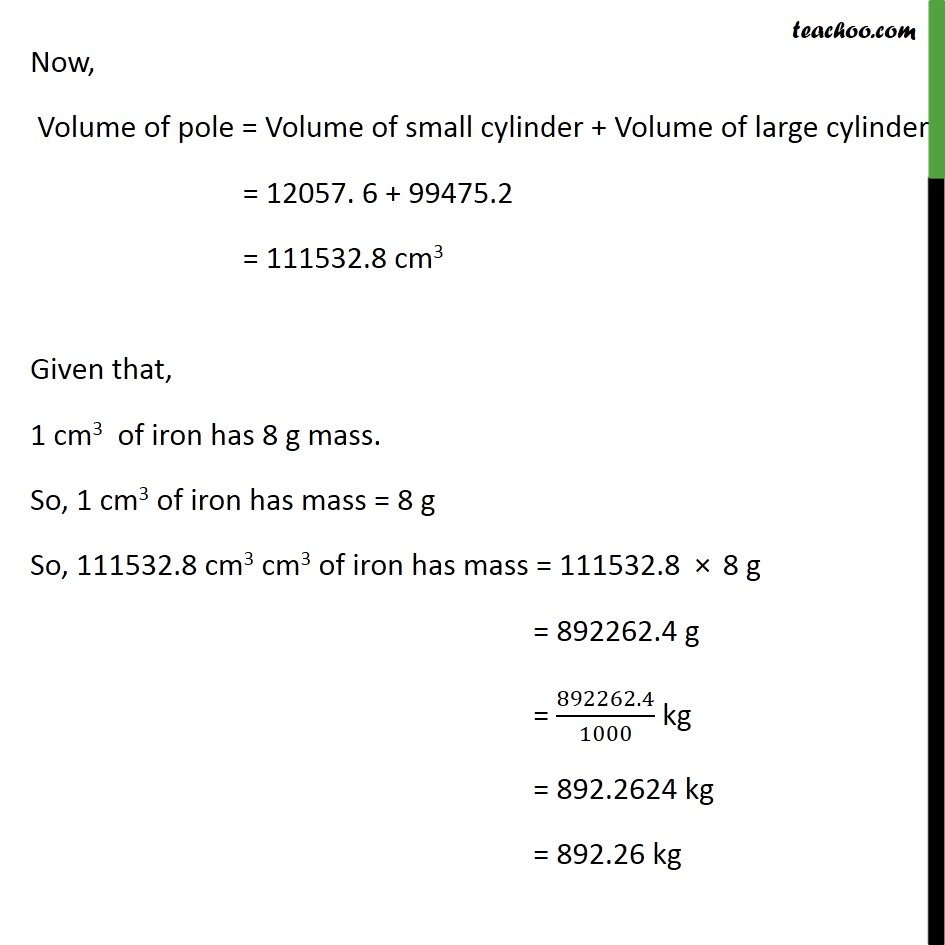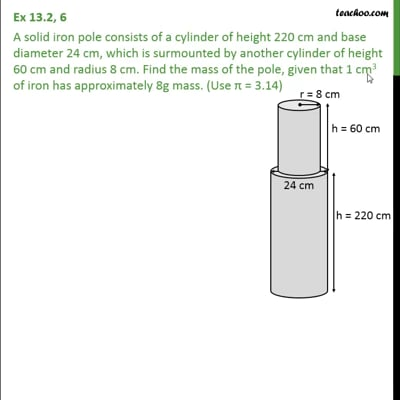Ex 13.2

Chapter 13 Class 10 Surface Areas and Volumes (Term 2)
Serial order wiseThis video is only available for Teachoo black users

### Transcript

Ex 13.2, 6 A solid iron pole consists of a cylinder of height 220 cm and base diameter 24 cm, which is surmounted by another cylinder of height 60 cm and radius 8 cm. Find the mass of the pole, given that 1 cm3 of iron has approximately 8g mass. (Use π = 3.14) To find mass of pole, we need to find volume of pole Volume of pole = volume of small cylinder + volume of large cylinder Volume of small cylinder Radius = r = 8 cm Height = h = 60 cm Volume of small cylinder = 𝜋𝑟2ℎ = 3.14×(8)2×(60) = 3.14×64×60 = 12057.6 cm2 Volume of large cylinder Height = h = 220 cm Radius = r = Diameter/2 = 24/2 = 12 cm Volume of large cylinder = 𝜋𝑟2ℎ = 3.14×(12)2×220 = 3.14×144×220 = 99475.2 cm3 Now, Volume of pole = Volume of small cylinder + Volume of large cylinder = 12057. 6 + 99475.2 = 111532.8 cm3 Given that, 1 cm3 of iron has 8 g mass. So, 1 cm3 of iron has mass = 8 g So, 111532.8 cm3 cm3 of iron has mass = 111532.8 × 8 g = 892262.4 g = 892262.4/1000 kg = 892.2624 kg = 892.26 kg